Next: Convolution end effects Up: FAMILIAR OPERATORS Previous: Zero padding is the

## Product of operators

We will look into details of Fourier transformation elsewhere. Here we use it as an example of any operator containing complex numbers. For now, we can think of Fourier transform as a square matrix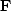.We denote the complex-conjugate transpose (or adjoint) matrix with a prime, i.e.,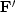.The adjoint arises naturally whenever we consider energy. The statement that Fourier transforms conserve energy iswhere.Substituting gives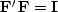which shows that the inverse matrix to Fourier transform happens to be the complex conjugate of the transpose of.

With Fourier transforms, zero padding and truncation are particularly prevalent. Most programs transform a dataset of length of 2n, whereas dataset lengths are often of length.The practical approach is therefore to pad given data with zeros. Padding followed by Fourier transformationcan be expressed in matrix algebra as(5)
According to matrix algebra, the transpose of a product, say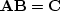,is the product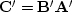in reverse order. So the adjoint program is given by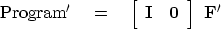(6)
Thus the adjoint program truncates the data after the inverse Fourier transform.Next: Convolution end effects Up: FAMILIAR OPERATORS Previous: Zero padding is the
Stanford Exploration Project
10/21/1998